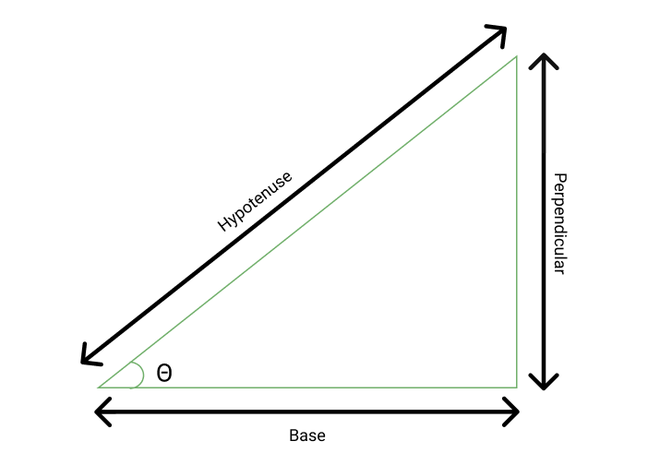GeeksforGeeks App
Open AppBrowser
Continue

# C# Program to Find the Value of Sin(x)

Sin(x) is also known as Sine. It is a trigonometric function of an angle. In a right-angled triangle, the ratio of the length of the perpendicular to the length of the hypotenuse is known as the sine of an angle.`sin θ = perpendicular / hypotenuse`

The values of sine of some of the common angles are given below,

1. sin 0°  = 0
2. sin 30° = 1 / 2
3. sin 45° = 1 / √2
4. sin 60° = √3 / 2
5. sin 90° = 1

This article focuses upon how we can calculate the sine of an angle by in C#.

### Method 1

We can calculate the sine of an angle by using the inbuilt sin() method. This method is defined under the Math class and is a part of the system namespace. Math class is quite useful as it provides constants and some of the static methods for trigonometric, logarithmic, etc.

Syntax:

public static double Sin (double angle);

Parameter:

• angle: A double value (angle in radian)

Return type:

• double: If “angle” is double
• NaN: If “angle” is equal to NaN, NegativeInfinity, or PositiveInfinity

Example 1:

## C#

 `// C# program to illustrate how we can``// calculate the value of sin(x)``// using Sin() method``using` `System.IO;``using` `System;` `class` `GFG{``    ` `static` `void` `Main()``{``    ` `    ``// Angle in degree``    ``double` `angleInDegree1 = 0;``    ` `    ``// Converting angle in radian``    ``// since Math.sin() method accepts``    ``// angle in radian``    ``double` `angleInRadian1 = (angleInDegree1 * (Math.PI)) / 180;``    ` `    ``// Using Math.Sin() method to calculate value of sine``    ``Console.WriteLine(``"The value of sin({0}) = {1} "``,``                      ``angleInDegree1, Math.Sin(angleInRadian1));``                      ` `    ``// Angle in degree``    ``double` `angleInDegree2 = 45;``    ` `    ``// Converting angle in radian``    ``// since Math.sin() method accepts``    ``// angle in radian``    ``double` `angleInRadian2 = (angleInDegree2 * (Math.PI)) / 180;``    ` `    ``// Using Math.Sin() method to calculate value of sine``    ``Console.WriteLine(``"The value of sin({0}) = {1} "``,``                      ``angleInDegree2, Math.Sin(angleInRadian2));``    ` `    ``// Angle in degree``    ``double` `angleInDegree3 = 90;``       ` `    ``// Converting angle in radian``    ``// since Math.sin() method accepts``    ``// angle in radian``    ``double` `angleInRadian3 = (angleInDegree3 * (Math.PI)) / 180;``    ` `    ``// Using Math.Sin() method to calculate value of sine``    ``Console.WriteLine(``"The value of sin({0}) = {1} "``,``                      ``angleInDegree3, Math.Sin(angleInRadian3));``    ` `    ``// Angle in degree``    ``double` `angleInDegree4 = 135;``       ` `    ``// Converting angle in radian``    ``// since Math.sin() method accepts``    ``// angle in radian``    ``double` `angleInRadian4 = (angleInDegree4 * (Math.PI)) / 180;``    ` `    ``// Using Math.Sin() method to calculate value of sine``    ``Console.WriteLine(``"The value of sin({0}) = {1} "``,``                      ``angleInDegree4, Math.Sin(angleInRadian4));``}``}`

Output

```The value of sin(0) = 0
The value of sin(45) = 0.707106781186547
The value of sin(90) = 1
The value of sin(135) = 0.707106781186548 ```

Example 2:

## C#

 `// C# program to illustrate how we can``// calculate the value of sin(x)``// using Sin() method``using` `System;` `class` `GFG{` `static` `public` `void` `Main()``{``    ` `    ``// Angle in radian``    ``double` `angle1 = Double.NegativeInfinity;``    ` `    ``// Angle in radian``    ``double` `angle2 = Double.PositiveInfinity;``    ` `    ``// Angle in radian``    ``double` `angle3 = Double.NaN;``    ` `    ``// Using Math.Sin() method to calculate value of sine``    ``Console.WriteLine(``"The value of sin({0}) = {1} "``,``                      ``angle1, Math.Sin(angle1));``    ` `    ``// Using Math.Sin() method to calculate value of sine``    ``Console.WriteLine(``"The value of sin({0}) = {1} "``,``                      ``angle2, Math.Sin(angle2));``    ` `    ``// Using Math.Sin() method to calculate value of sine``    ``Console.WriteLine(``"The value of sin({0}) = {1} "``,``                      ``angle3, Math.Sin(angle3));``}``}`

Output

```Sine of angle1: NaN
Sine of angle2: NaN
Sine of angle3: NaN```

Time complexity: O(n), where n is the number of terms calculated for the Maclaurin’s series approximation of sin(x).

Space complexity: O(1).

### Method 2

We can calculate the value of sine of an angle using Maclaurin expansion. So the Maclaurin series expansion for sin(x) is:

`sin(x) = x - x3 / 3! + x5 / 5! - x7 / 7! + ....`

Follow the steps given below to find the value of sin(x):

1. Initialize a variable angleInDegree that stores the angle (in degree) to be calculated.
2. Initialize another variable terms that stores the number of terms for which we can approximate the value of sin(x).
3. Declare a global function findSinx.
4. Declare a variable current. It stores the angle in radians.
5. Initialize a variable answer with current. It will store our final answer.
6. Initialize another variable temp with current.
7. Iterate from i = 1 to i = terms. At each step update temp as temp as ((-temp) * current * current) / ((2 * i) * (2 * i + 1)) and answer as answer + temp.
8. Eventually, return the answer from findSinX function.

This formula can compute the value of sine for all real values of x.

Example:

## C#

 `// C# program to illustrate how we can``// calculate the value of sin(x)``// using Maclaurin's method``using` `System;` `class` `GFG{` `static` `double` `findSinX(``int` `angleInDegree, ``int` `terms)``{``    ` `    ``// Converting angle in degree into radian``    ``double` `current = Math.PI * angleInDegree / 180f;``    ` `    ``// Declaring variable to calculate final answer``    ``double` `answer = current;``    ``double` `temp = current;``    ` `    ``// Loop till number of steps provided by the user``    ``for``(``int` `i = 1; i <= terms; i++)``    ``{``        ` `        ``// Updating temp and answer accordingly``        ``temp = ((-temp) * current * current) /``                    ``((2 * i) * (2 * i + 1));``        ``answer = answer + temp;``    ``}` `    ``// Return the final answer``    ``return` `answer;``}` `// Driver code``static` `public` `void` `Main()``{``    ` `    ``// Angle in degree``    ``int` `angleInDegree1 = 45;``  ` `    ``// Number of steps``    ``int` `terms1 = 10;` `    ``// Calling function to calculate sine of angle``    ``double` `answer1 = findSinX(angleInDegree1, terms1);` `    ``// Print the final answer``    ``Console.WriteLine(``"The value of sin({0}) = {1}"``,``                      ``angleInDegree1, answer1);``    ` `    ``// Angle in degree``    ``int` `angleInDegree2 = 90;``    ` `    ``// Number of steps``    ``int` `terms2 = 20;` `    ``// Calling function to calculate sine of angle     ``    ``double` `result2 = findSinX(angleInDegree2, terms2);` `    ``// Print the final answer``    ``Console.WriteLine(``"The value of sin({0}) = {1}"``,``                      ``angleInDegree2, result2);``    ` `    ``// Angle in degree``    ``int` `angleInDegree3 = 135;` `    ``// Number of steps``    ``int` `terms3 = 30;` `    ``// Calling function to calculate sine of angle         ``    ``double` `result3 = findSinX(angleInDegree3, terms3);` `    ``// Print the final answer``    ``Console.WriteLine(``"The value of sin({0}) = {1}"``,``                      ``angleInDegree3, result3);``    ` `    ``// Angle in degree``    ``int` `angleInDegree4 = 180;``  ` `    ``// Number of steps``    ``int` `terms4 = 40;``    ` `    ``// Calling function to calculate sine of angle``    ``double` `result4 = findSinX(angleInDegree4, terms4);` `    ``// Print the final answer``    ``Console.WriteLine(``"The value of sin({0}) = {1}"``,``                      ``angleInDegree4, result4);``}``}`

Output

```The value of sin(45) = 0.707106781186547
The value of sin(90) = 1
The value of sin(135) = 0.707106781186548
The value of sin(180) = 2.34898825287367E-16```

Time complexity: O(n). //n is the number of terms passed as input.

Space complexity: O(1).

My Personal Notes arrow_drop_up